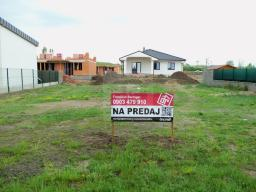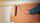# Property

The length of the rectangle-shaped property is 8 meters less than three times of the width. If we increase the width 5% of a length and lendth reduce by 14% of the width it will increase the property perimeter by 13 meters. How much will the property cost if per 1 square meter we will pay € 8.6?

x =  12235908

### Step-by-step explanation:

a = 3b - 8
2[(b + 0.05a) + (a-0.14 b)] = 2(a+b)+13
x = 8.6 • 2062 • 690

a = 3•b - 8
2•((b + 0.05•a) + (a-0.14•b)) = 2•(a+b)+13
x = 8.6 • 2062 • 690

a-3b = -8
0.1a-0.28b = 13
x = 12235908

a = 2062
b = 690

Our linear equations calculator calculates it.Did you find an error or inaccuracy? Feel free to write us. Thank you!Tips to related online calculators
Do you have a linear equation or system of equations and looking for its solution? Or do you have a quadratic equation?
Do you want to convert length units?

#### You need to know the following knowledge to solve this word math problem:

We encourage you to watch this tutorial video on this math problem:

## Related math problems and questions:

• PlotThe length of the rectangle is 8, smaller than three times the width. If we increase the width by 5% of the length and the length is reduced by 14% of the width, the circumference of the rectangle will be increased by 30 m. What are the dimensions of the
• Rectangular gardenThe perimeter of Peter's rectangular garden is 98 meters. The width of the garden is 60% shorter than its length. Find the dimensions of the rectangular garden in meters. Find the garden area in square meters.
• Area of gardenIf the width of the rectangular garden is decreased by 2 meters and its length is increased by 5 meters, the area of the rectangle will be 0.2 ares larger. If the width and the length of the garden will increase by 3 meters, its original size will increas
• PainterHow many euros we will pay for repainting the room shaped cuboid with a length of 4.5 meters, width of 2.5 meters and a height of 3 meters, if for 1 m2 with paint we pay € 1.5?
• The roomThe room has a cuboid shape with dimensions: length 50m and width 60dm and height 300cm. Calculate how much this room will cost paint (a floor is not painted) if the window and door area is 15% of the total area and 1m2 cost 15 euro.
• Rectangle fieldThe field has a shape of a rectangle having a length of 119 m and a width of 19 m. , How many meters have to shorten its length and increase its width to maintain its area and circumference increased by 24 m?
• Square sideIf we enlarge the square side a = 5m, its area will increase by 10,25%. How many percent will the side of the square increase? How many percent will it increase the circumference of the square?
• Square - increased perimeterHow many times is increased perimeter of the square, where its sides increases by 150%? If the perimeter of square will increase twice, how much% increases the content area of the square?
• RectangleThe width of the rectangle is 65% of its length. Perimeter of the rectangle is 132 cm. Determine the dimensions of the rectangle.
• RectangleFind the dimensions of the rectangle, whose perimeter is 108 cm and the length is 25% larger than the width.
• Three shapes1/5 of a circle is shaded. The ratio of area if square to the sum of area of rectangle and that of the circle is 1:2. 60% of the square is shaded and 1/3 of the rectangle is shaded. What is the ratio of the area of circle to that of the rectangle?
• RectangleThe length of the rectangle is 12 cm greater than 3 times its width. What dimensions and area this rectangle has if ts circumference is 104 cm.
• PaintingThe room is long 50 meters and wide 60dm and 300 cm high. Calculate how much it will cost painting if area of windows and doors is 15% of the total area. One square meter cost 50cents.
• Percent changeIf the length of a rectangle is increased by 25% and the width is decreased by 10%, the area of the rectangle is larger than the area of the original rectangle by what percent?
• Dimensions 3A perimeter of a rectangular field is 96 meters. The length is four meters less than three times the width. Find the length and width (its dimensions).
• The rectangleDetermine the area of the rectangle where the length and width are in the ratio 5:2 and its length is 7.5 cm longer than its width. Determine also its length and its width.
• The hallThe hall had a rectangular ground plan one dimension 20 m longer than the other. After rebuilding the length of the hall declined by 5 m and the width has increased by 10 m. Floor area increased by 300 m2. What were the original dimensions of the hall?